output.to from Sideway

Draft for Information Only

# Content

```  Sound Wave    Wave Propagation    Speed of Sound   Frequency and Wavelength```

## Sound Wave

One feature that associated with sound is the vibration of medium particles. The vibration is a local displacement of medium particle. Energy of the vibrating particle will transfer to its neighborhood during vibrating. Acoustic disturbance propagate through particle displacement, the particle will return to its former state after the disturbance has passed. The acoustic energy is then travels through the medium in form of wave,.

### Wave Propagation

The two common modes of sound wave propagation are longitudinal wave and transverse wave.

For longitudinal wave, the wave propagation is along with or parallel to the direction of medium particle vibration.

For transverse wave, the wave propagation is at right to the direction of medium particle vibration.

In fluid, for example air, water, sound wave travels in the form of longitudinal wave. The displacement of medium particle is a  series of  alternating local compression followed by a local rarefaction and causing alternating pressure variations from the ambient pressure.

In solid, for example metal, concret, sound wave travels in the form of both longitudinal and transverse wave. The longitudinal wave propagation in solid is the same as in fluid. But the transverse wave caused by the displacement of medium particle at right angle to the wave propagation is due to a series of alternating shear stress resulting from the displacement of neighborhood medium particles.

### Speed of Sound

The speed of sound in a medium mainly depends on the medium and the temperature of medium. By general assumption, the speed of sound can be simplified to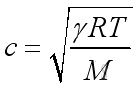where

c is speed of sound γ is (specific heat at constant pressure/specific heat at constant volume) = 1.4 for air,
R is gas constant = 8317 m2/(s2 oK),br /> T is temperature (oK),br /> M is molecular weight of gases

Therefore the speed of sound in air can be calculated by :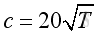.

where

T is in Kelvin and T=273.2o+oC
and in room temperature,  T=20oC, imply c=340 m/s

Some sound speed under room temperature and atmosphere pressure:

Medium Longitudinal Wave Transverse Wave
Air 340
Water 1500
Aluminum 6400 3040
Steel 5900 3200
Copper 4700 2110
Quartz 5700 3920
Heavy Concrete 3350 2225

### Frequency and Wavelength

Frequency and wavelength are the two basic characteristics of a sound wave. Amplitude is the magnitude of a sound wave related to the loudness of a sound. Frequency is the number of wave oscillations per unit time related to the pitch of a sound.

Frequency, ƒ (Hz or cycle per sec): Number of pressure fluctuations per second.

Period, T (sec. per cycle): Duration of one pressure fluctuation cycle.

Therefore,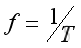.

Angular frequency, ω (Rad per sec): Or circular frequency, in term of rotation rate.

Therefore,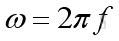.

Wavelength, λ (m): The number of circular wavelengths per unit distance.

Therefore,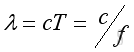.

Wave Number, k (1/m): Distance traveled by the sound in one period, T.

Therefore,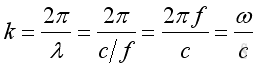.References

1. Michael P. Norton; Denis G. Karczub,, 2003, Fundamentals of Noise and Vibration Analysis for Engieer, Cambridge, United Kingdom
2. G. Porges, 1977, Applied Acoustics, Edward Arnold Limited, Britain
3. Conrad J. Hemond, 1983, Engineering Acoustics & Noise Control, Prentice-Hall, USA
4. F. Fahy, 2001, Foundations of Engineering Acoustics, Academic Press, UKID: 100900007 Last Updated: 9/5/2010 Revision: 0 Ref:Home (5)

Management

HBR (3)

Information

Recreation

Hobbies (7)

Culture

Chinese (1097)

English (336)

Reference (66)

Computer

Hardware (149)

Software

Application (187)

Digitization (24)

Numeric (19)

Programming

Web (618)CSS (SC)

ASP.NET (SC)

HTML

Knowledge Base

Common Color (SC)

Html 401 Special (SC)

OS (388)MS Windows

Windows10 (SC)

.NET Framework (SC)

DeskTop (7)

Knowledge

Mathematics

Formulas (8)

Number Theory (206)

Algebra (20)

Trigonometry (18)

Geometry (18)

Calculus (67)

Complex Analysis (21)

Engineering

Tables (8)

Mechanical

Mechanics (1)

Rigid Bodies

Statics (92)

Dynamics (37)

Fluid (5)

Control

Acoustics (19)

Biology (1)

Geography (1)